Your data analysis solution

# Gaussian mixture model clustering in Excel tutorial

2017-10-20

This tutorial will help you set up and interpret a Gaussian Mixture Model (GMM) in Excel using the XLSTAT software.
Not sure if this is the right clustering feature you need? Check out this guide.

## Gaussian mixture models for clustering

These models are commonly used for a clustering purpose. They can provide a framework for assessing the partitions of the data by considering that each component represents a cluster. These models have two main advantages:

• It is a probabilistic method for obtaining a fuzzy classification of the observations. The probability of belonging to each cluster is calculated and a classification is usually achieved by assigning each observation to the most likely cluster. These probabilities can also be used to interpret suspected classifications.
• Mixture modeling is very flexible.

## Dataset for Gaussian mixture model

The data correspond to the famous iris of Fisher presented in [Fisher, R. A. (1936), The use of multiple measurements in taxonomic problems. Annals of Eugenics, 7, Part II, 179–188]

These data gives the measurements (in centimeters) of the petal length and width, for 150 flowers of 3 species of iris (setosa, versicolor, and virginica).

An Excel sheet containing both the data and the results for use in this tutorial can be downloaded by clicking here.

The aim is to fit a Gaussian mixture model and recover the data structure in three clusters.

## Setting up a Gaussian mixture model

After opening XLSTAT, select the XLSTAT / Analyzing data / Gaussian mixture models command, or click on the corresponding button of the Analyzing data toolbar.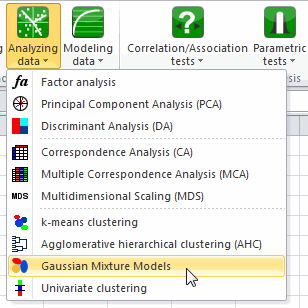Once you've clicked on the button, the dialog box appears.

The data are presented in a table of 150 rows and 2 columns. It is assumed that the labels are unknown and that the weight of each row is the same. As the classification of the data is done according to the length and width of the iris petal, the option Multidimensional is chosen.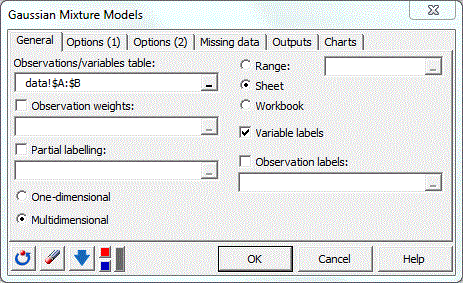In the Options(1) tab, three inference algorithms with four selection criteria and three methods of initialization are proposed. The user can also set the maximum number of iterations of the inference algorithm and its convergence threshold. Here, we choose a random initialization with two replicates and leave all the other options to their default values.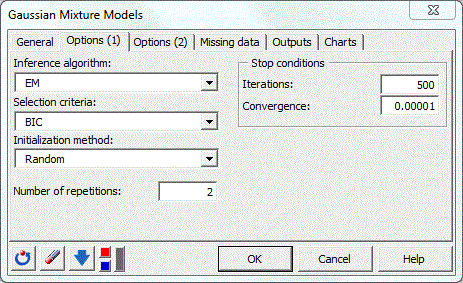In the Options(2) tab,  a list of all the Gaussian mixture models is available. The maximum and minimum number of classes can be modified and the mixture proportions can be forced to be equal. Here, we choose to test the EEE and EEV models for a number of classes which varies from 2 to 5.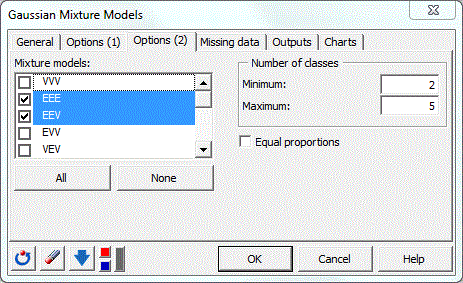The computations begin once you have clicked on OK. The results will then be displayed in a new sheet.

## Interpreting the results of a gaussian mixture model clustering

The first results displayed are the statistics for the various varaibles (length and width). Next, the value of the selection criterion for all models and for a number of classes which varies from 2 to 5 are displayed.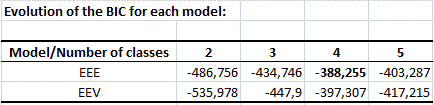Then the estimated parameters of the selected model are given (proportions, means and variances).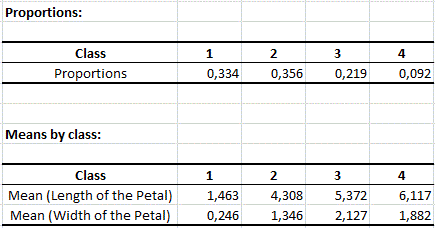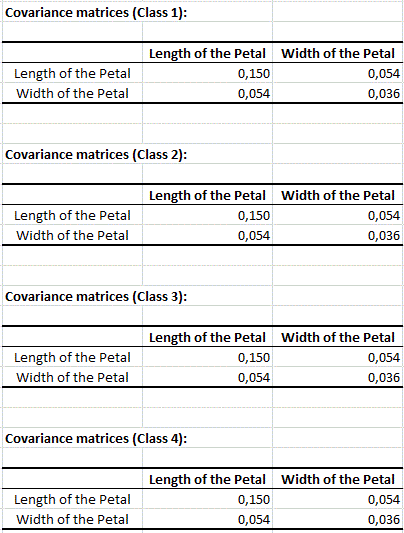A table displaying the characteristics of the selected model is then presented (BIC, AIC, log likelihood, NEC, ...).

In the next table the results in terms of probability estimation and classification for the first observations of the data set available are showed. The classification is computed according to the probabilities via the MAP (Maximum A Posteriori) rule. We can see that 3 classes have been selected.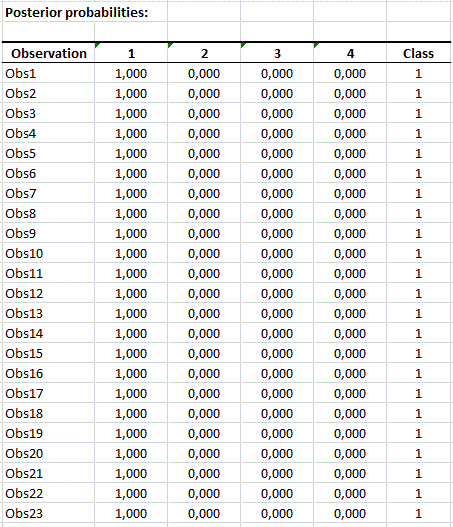Finally, a graph of the clustered data is provided.Many other features and options are available in the mixture models with XLSTAT including observation weights, partial labeling, 14 inference algorithm...

### Contact Us

https://cdn.desk.com/
false
desk
Loading
seconds ago
a minute ago
minutes ago
an hour ago
hours ago
a day ago
days ago
about
false
Invalid characters found
/customer/portal/articles/autocomplete
9283